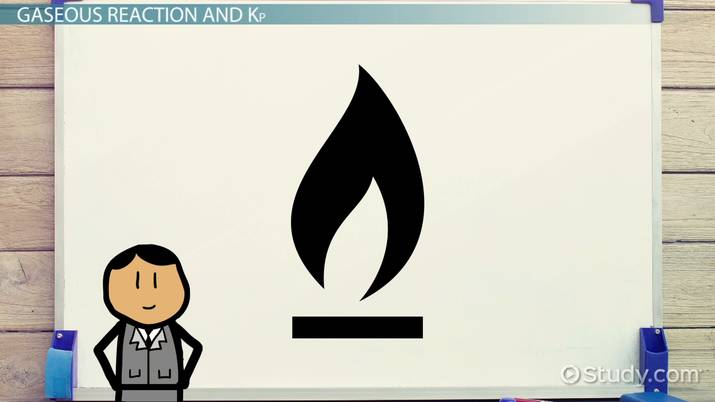# Law of Mass Action: Definition, Application & Equation

Lesson Transcript
Instructor: Saranya Chatterjee

Saranya has a masters degree in Chemistry and in Secondary Education. She has taught high school, AP chemistry for 2 years and is teaching undergraduate college chemistry for 3 years.

This lesson will discuss law of mass action. It will derive the equations between equilibrium constants at constant partial pressure (Kp), concentrations (Kc) and discuss some real-life applications of chemical equilibrium.

## What Is Law of Mass Action?

Imagine the flow of water from a high level to a lower level. Will the water flow forever? No. The flow will stop when both levels become equal. This phenomenon may be considered a state of equilibrium. This idea applies in chemical reactions as well.

In chemistry, an active mass is the mass of a substance that is actually reacting. The law of mass action states that, at a constant temperature, the rate of a chemical reaction is proportional to the active masses of the reacting substances. Let us consider the following reaction:

A+B → C+D

Let C' denote the concentrations of the components at a given instant during the reaction process. Let the concentrations of A, B, C and D be C'A, C'B, C'C and C'D. According to the law, the rate of reaction (RAB) between A and B at that moment will be:

RAB = k1 C'A C'B where k1, the constant of proportionality, depends upon reactants and temperature.

During the reaction, the concentrations of the reactants A and B would diminish, and therefore its rate would also diminish with time. Similarly, the rate of the opposite reaction (RCD) between C and D at that moment will be:

RCD = k2 C'C C'D

Since the concentrations of C and D would increase, the rate RCD would also increase. A time will come when the rates of the reaction in the two opposite directions will be equal; that is, RAB = RCD. The system will then attain equilibrium and there will be no further change in the masses of the components of the system. At equilibrium, let the concentrations be expressed by C (instead of C'). Then:

• RAB = RCD
• k1 CA CB = k2 CC CD
• CC CD / CA CB = k1 / k2 = Kc (constant)

Kc is the equilibrium constant of the reaction.An error occurred trying to load this video.

Try refreshing the page, or contact customer support.

Coming up next: Equilibrium Constant (K) and Reaction Quotient (Q)

### You're on a roll. Keep up the good work!

Replay
Your next lesson will play in 10 seconds
• 0:04 What is the Law of…
• 2:40 Multiple Molecules
• 3:49 Gaseous Reaction and KP
• 5:46 Applications in Problems
• 7:29 Real Life Applications
• 8:02 Lesson Summary
Save Save

Want to watch this again later?

Timeline
Autoplay
Autoplay
Speed Speed

## Multiple Molecules

Okay, but what happens if multiple molecules of the reactants are involved? Say, 2A + 3B = C + 2D. The rates are given by:

• RAB = k1 C2A C3B
• RCD = k2 CC C2D

At equilibrium, k1 C2A C3B = k2 CC C2D

So, Kc = k1 / k2 = CC C2D / CC C2D

That is, the coefficients in the stoichiometric equation become powers of the concentrations in the expression of equilibrium constant. In general, for a reversible reaction of the type like that shown here -

aA + bB + cC + ..... → dD + eE + fF + .......

- the equilibrium constant, Kc, would look like this:

Kc = Cd D Ce E Cf F...../ Ca A Cb B Cc C....

## Gaseous Reaction and Kp

In a gaseous reaction, components are usually expressed in terms of their partial pressures instead of molarities. If the gases are supposed to behave ideally, partial pressures are expressed as PA, PB, ...PD, PE and so on.

Then the total pressure, P = PA + PB + .. + PD PE + ....

The equation for Kp looks like this, where Kp is the equilibrium constant of a reaction found by adding the partial pressures:

Kp = PdD * PeE...../ PaA PbB ....

Again, assuming ideality, we can express pressures as:

PA = CA RT, and PB = CB RT

Therefore, the equation for the equilibrium constant looks like this:

Kp = (CD RT)d * (CE RT)e * ..../ (CA RT)a * (CB RT)b

= {(CD)d * (CE)e *.../ (CA)a * (CB)b} * (RT) d+e + ... -a-b...

So, Kp = Kc (RT) Δn

where Δn = number of product molecules minus number of reactant molecules. This is a relation between the equilibrium constants derived from partial pressure units and from molar concentrations unit of components.

To unlock this lesson you must be a Study.com Member.

### Register to view this lesson

Are you a student or a teacher?